Question

electromagnetic

As an electrical engineer, you are required to construct a bounce diagram for a lossless type of transmission lines as in Figure 3.1 to be operated in a frequency, ??=1 GHz. The transmission line is constructed as two parallel wires (Figure 3.2) with diameter, ??=2?? (unit in mm) and separated from center to center by distance, ?? (unit in mm). The relative permittivity, ???? is 2, and permeability, ??=????. The length, ?? of the lines is 100 m. Given ????=0.5????, ????=2????, and ????=12 ??. In the bounce diagram, show the transient voltage value at the load and generator ends for 0<? <7? 1, where ? 1=??/?? and ??= wave or phase velocity. (10 marks)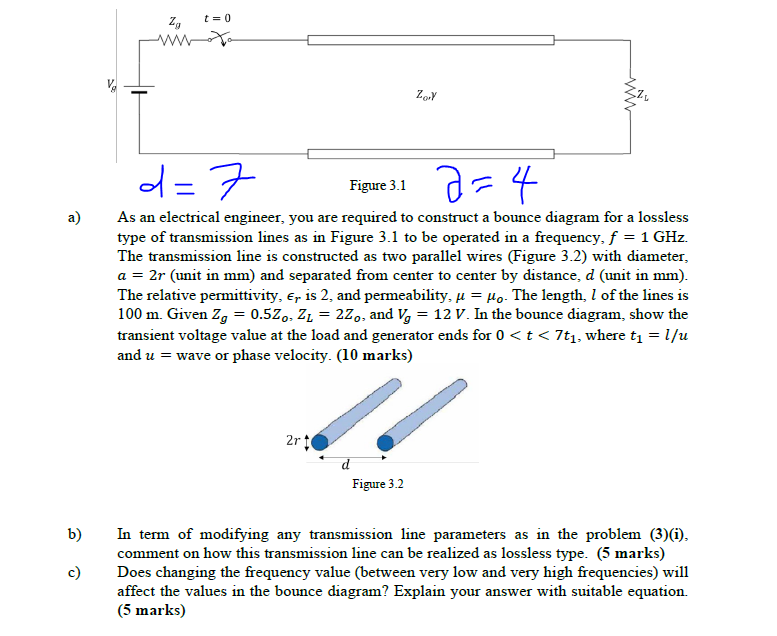We need at least 9 more requests to produce the answer.

1 / 10 have requested this problem solution

The more requests, the faster the answer.

All students who have requested the answer will be notified once they are available.

Earn Coins

Coins can be redeemed for fabulous gifts.

Similar Homework Help Questions
• A coaxial line with cylindrical symmetry as shown in cross section by Figure A1-1. It is...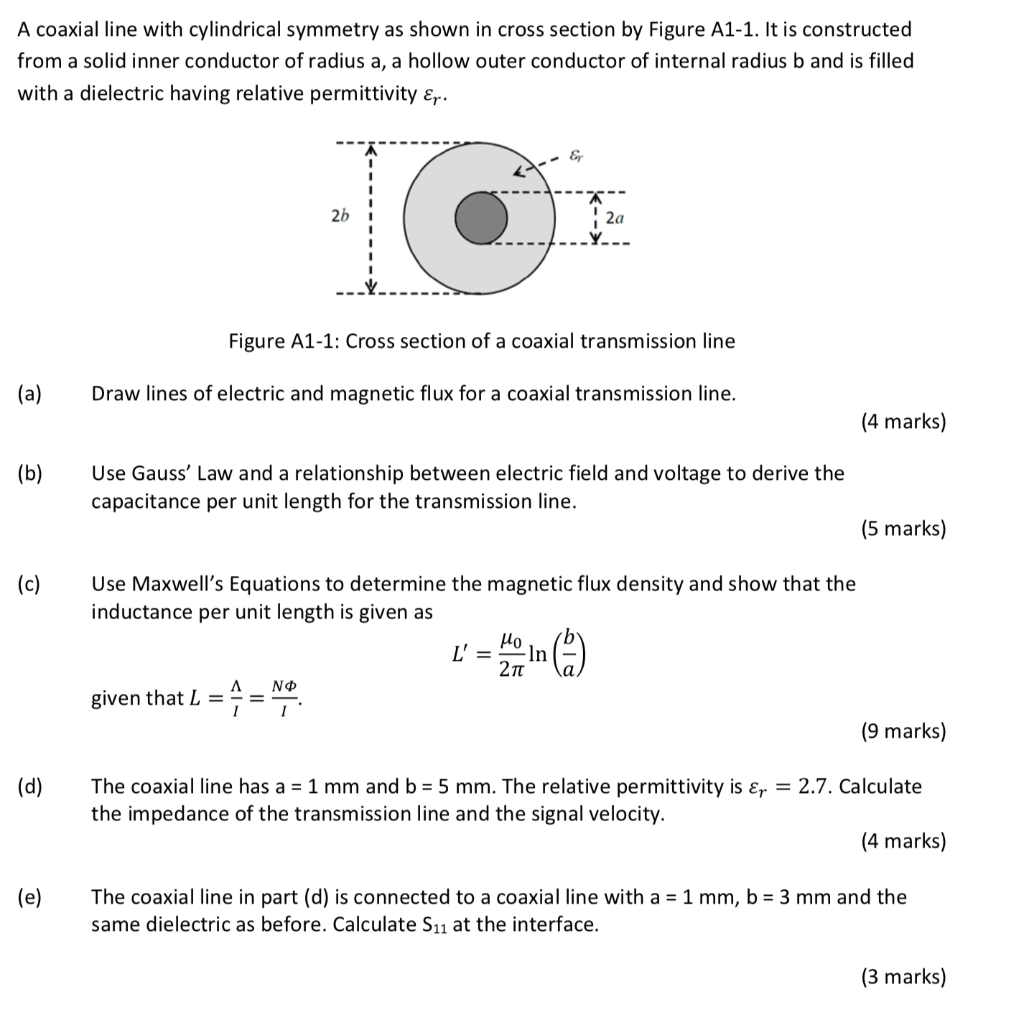A coaxial line with cylindrical symmetry as shown in cross section by Figure A1-1. It is constructed from a solid inner conductor of radius a, a hollow outer conductor of internal radiusb and is filled with a dielectric having relative permittivity & 2b 2a Figure A1-1: Cross section of a coaxial transmission line (a) Draw lines of electric and magnetic flux for a coaxial transmission line. (4 marks) (b) Use Gauss' Law and a relationship between electric field and voltage...

• note that 1) single phase system 2) base values at transmission line circuit A 100 MVA,...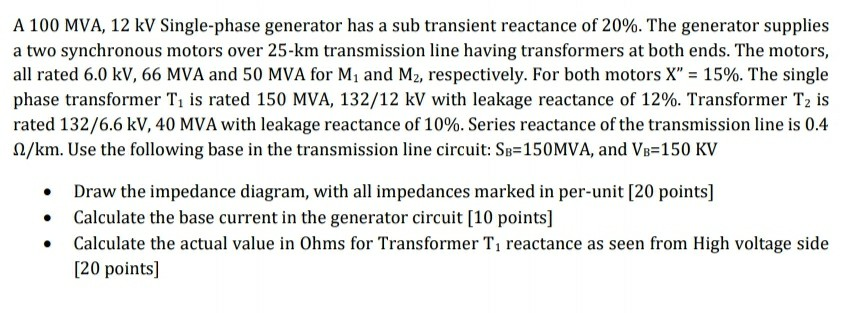note that 1) single phase system 2) base values at transmission line circuit A 100 MVA, 12 kV Single-phase generator has a sub transient reactance of 20%. The generator supplies a two synchronous motors over 25-km transmission line having transformers at both ends. The motors, all rated 6.0 kV, 66 MVA and 50 MVA for Mi and M2, respectively. For both motors X" = 15%. The single phase transformer T is rated 150 MVA, 132/12 kV with leakage reactance of...

• Figure 1 shows a single-line diagram of a three phase, 60-Hz, synchronous generator, connected th...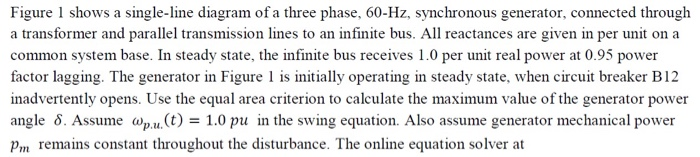Figure 1 shows a single-line diagram of a three phase, 60-Hz, synchronous generator, connected through a transformer and parallel transmission lines to an infinite bus. All reactances are given in per unit on a common system base. In steady state, the infinite bus receives 1.0 per unit real power at 0.95 power factor lagging. The generator in Figure 1 is initially operating in steady state, when circuit breaker B12 inadvertently opens. Use the equal area criterion to calculate the maximum...

• Question 2 A 60 Hz synchronous generator with Xd 0.2 pu transient reactance is connected to...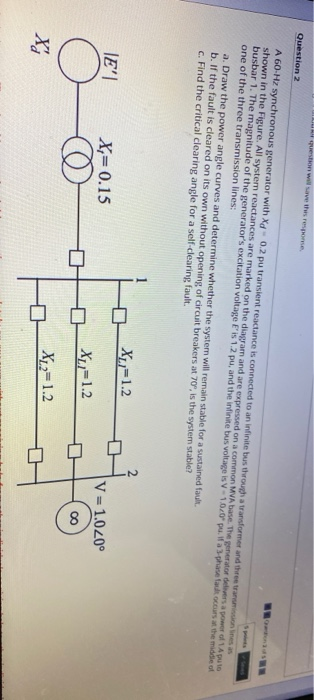Question 2 A 60 Hz synchronous generator with Xd 0.2 pu transient reactance is connected to an infinite us through a transformer and the transmisiones as shown in the Figure. All system reactances are marked on the diagram and are expressed on a common MVA base. The generator dies a power of Auto busbar 1. The magnitude of the generator's excitation voltage e'is 12 pu, and the infinite bus voltage is -1.00u. If a 3-phase fault occurs at the middle...

• Figure 1 Single line diagram b2 b3 b1 b4 grid Τι 13 A power system single line diagram is shown...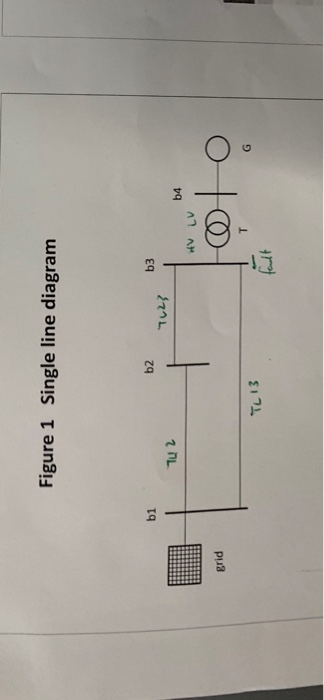Figure 1 Single line diagram b2 b3 b1 b4 grid Τι 13 A power system single line diagram is shown in Figure 1. The single line diagram shows a synchronous generator G connected to a large 50 Hz grid via its unit transformer T and a network of three transmission lines. Relevant details of the grid, transformer, generator and overhead lines are provided in Tables I,II,II & IV respectively. A double line to ground fault occurs at bus 3 Questions....

• Power system analysis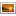Figure shows a single-line diagram of a three-phase, 60-Hz synchronous generator, connected through a transformer and parallel transmission lines to an infinite bus. All reactances are given in perunit on a common system base. If the infinite bus receives 1.0 per unit real power at 0.95 p.f. lagging. If circuit breakers B13 and B22 are opened after three cycles and then reclosed when the power angle reaches 35°.123.png Assume ωpu(t) = 1.0 pu in the swing equation. Assume that the temporary...

• The one-line diagram of a three-bus power system is shown in Figure 4. All impedances are...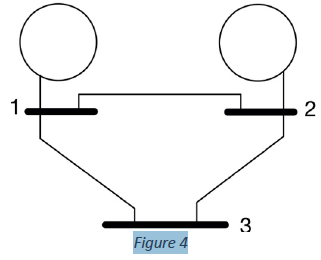The one-line diagram of a three-bus power system is shown in Figure 4. All impedances are expressed in per unit on a common MVA base. All resistances and shunt capacitances are neglected. Information on each component in this system is given below: • Each generator is represented by an emf behind the sub-transient reactance of j0.045 and their neutrals are connected to the ground. • Line 1-2 has reactance of j0.88 • Line 2-3 has reactance of j0.65 • Line...

• Obiective To investigate and do analysis on what happens before, during and after resonance in a...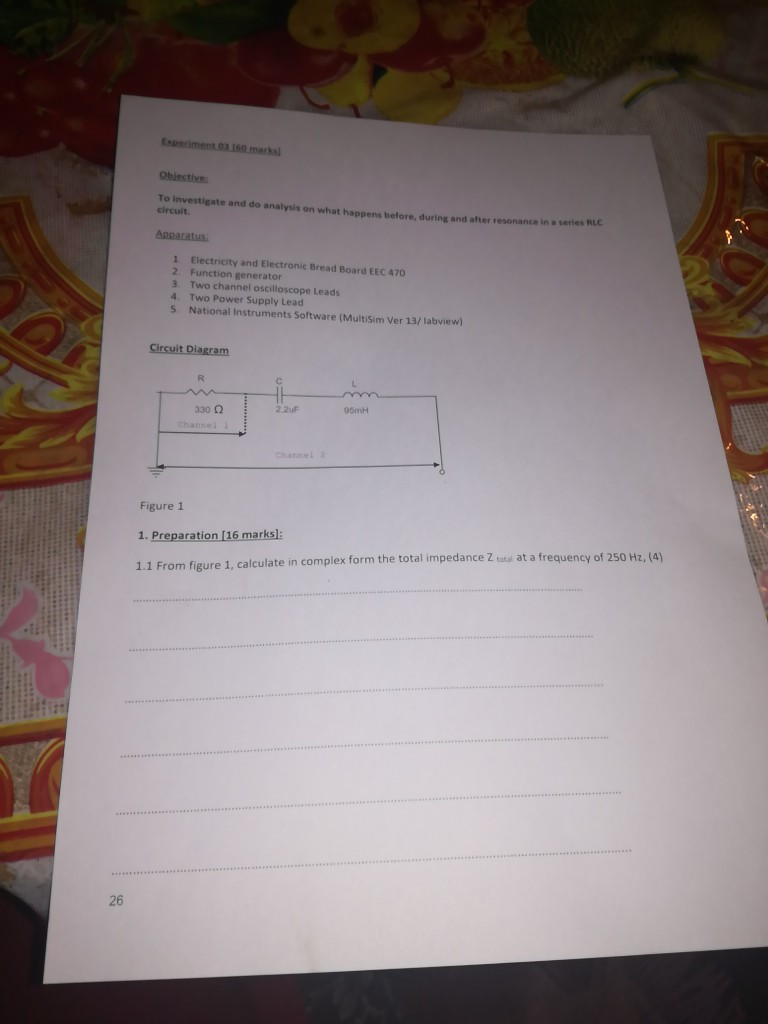Obiective To investigate and do analysis on what happens before, during and after resonance in a series circuit Apparatus 1 Electricity and Electronic Bread Board EEC 470 2 Function generator 3. Two channel oscilloscope Leads 4. Two Power Supply Lead 5 National Instruments Software (MultiSim Ver 13/labview) Circuit Diagram 330 95mH Figure 1 1. Preparation (16 marks]: 1.1 From figure 1. calculate in complex form the total impedance Z total at a frequency of 250 Hz, (4) 11.1 Draw waveforms...

• Please help me to develop an impedence bus matrix of zero sequence, positive sequence and negative...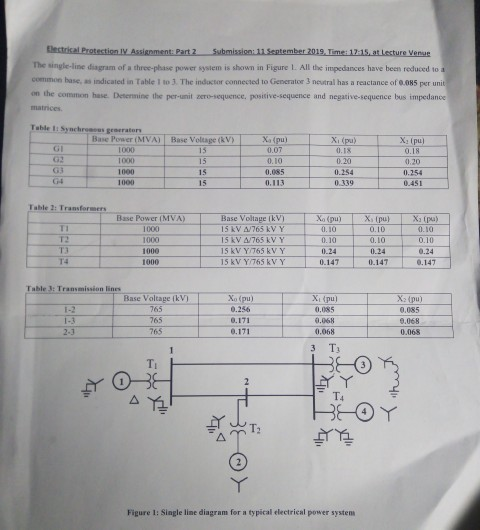Please help me to develop an impedence bus matrix of zero sequence, positive sequence and negative sequence network. thectical Protection IV Assignment: Part 2 Submission: 11 September 2019. Time: 17:15 a lecture Venue The ingle-line diagram of a three-phase power system is shown in Figure 1. All the impedances have been reduced to como base, as indicated in Table 1 to 3 The inductor connected to Generator 3 neutral has a reactance of 6.085 per unit the common hue. Determine...

• A single line diagram of a power system is shown in Fig. 2. The system data with equipment ratings and assumed sequence reactances are given the following table. The neutrals of the generator and A-Y...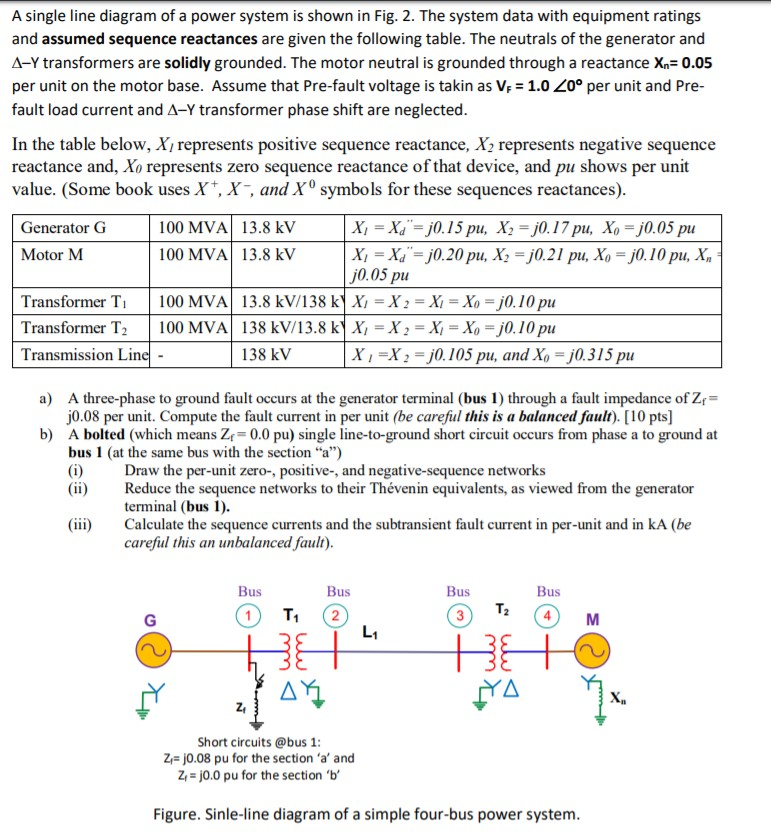A single line diagram of a power system is shown in Fig. 2. The system data with equipment ratings and assumed sequence reactances are given the following table. The neutrals of the generator and A-Y transformers are solidly grounded. The motor neutral is grounded through a reactance Xn 0.05 per unit on the motor base. Assume that Pre-fault voltage is takin as VF-1.0 ,0° per unit and Pre- fault load current and Δ-Y transformer phase shift are neglected In the...